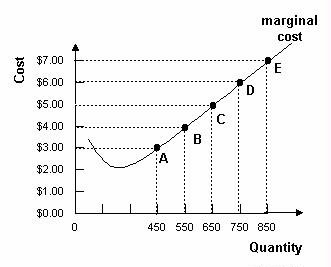### CFA Practice Question

There are 539 practice questions for this study session.

### CFA Practice Question

Refer to the graph below. If market price decreases from \$7.00 per unit to \$6.00 per unit, a profit-maximizing firm will ______.A. increase output from 650 to 750.
B. decrease output from 850 to 750.
C. produce 850 units of output.

The firm maximizes profit by setting price equal to marginal cost.